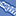# App Development

Announcements
Qlik Insider: SaaS capabilities for Data Integration and Analytics, May 25th! Register Now
cancel
Showing results for
Did you mean:Contributor II

## Get field value in color expression statement

Let's say I am developing a sheet and on that sheet I am using a table visualization. In this table, I add a calculated field, let's say it's a calculated measure. I enter my expression and I see my measure being calculated accordingly. That's all fine.

Now, for an example I want to be able to color code the field based on its value. The only way I know how to do this is to put the exact same formula in the color expression statement, surrounded by some IFs. This totally works, but it has a few problems:

• If I have a 3 way conditional (or otherwise need to reference the calculated value in more than one spot), then I have to insert the entire field expression (and it can get long) each time that I need it
• I have two places to update now - if I need to modify the set analysis of the field calculation, I have to now modify the corresponding set analysis of the color calculation
• It's not very scalable - if I want multiple fields to have their color code based on the same criteria, then if I ever want to extend that color coding criteria to other calculated fields, or if I need to consistently modify the criteria across multiple fields, it is not a matter of cut and paste on that field, but I have to modify around the calculation

Is there a way that I can just reference the calculated field's value in the color expression? Something like:

`=if(\$value > 0, green(), if(\$value < 0, red(), black())`

And to be clear, this would be helpful for more than just the foreground color. It would also be useful for the background color and the URL link text for items that I display as URLs.

Labels (4)

• ### value

1 Solution

Accepted SolutionsMVP

Have you seen the Column() function? I think this might be what you are looking for -> Column

4 RepliesMVP

Have you seen the Column() function? I think this might be what you are looking for -> ColumnContributor II
Author

Hi @sunny_talwar, yes, this Column() function is significantly more scalable and solves most of the problems that I listed in my three bullet points of my original post. A few comments though:

• The Column() function only works on measures. What I described in my original post was a measure, however, I have run into scenarios where it would be helpful to do the same operation on dimensions, since I often also have calculated dimensions too
• The Column() function takes a single argument, which requires me to know the order of my measures. Of course, this should never be too big of an issue since I am always the author of the entire table, but if I reorder the columns, and two measures switch order priority, then I now have to update the argument in the Column() function
• Because the Column() function requires a single argument, I still can't copy and paste a color formula between multiple fields like I could if there were just a \$value construct that I could leverage

With all of this in mind, is there also a function that you know of that addresses some of the challenges I listed above? (If not, the Column() function gets me 65% of the way there.)MVP

@mplautz wrote:
• The Column() function only works on measures. What I described in my original post was a measure, however, I have run into scenarios where it would be helpful to do the same operation on dimensions, since I often also have calculated dimensions too

May be use GetObjectField for this one GetObjectField

@mplautz wrote:
• The Column() function takes a single argument, which requires me to know the order of my measures. Of course, this should never be too big of an issue since I am always the author of the entire table, but if I reorder the columns, and two measures switch order priority, then I now have to update the argument in the Column() function

You can address this by using Expression label instead of Column() function. So if you expression is labeled XYZ... you can use [XYZ] as the column reference

@mplautz wrote:
• Because the Column() function requires a single argument, I still can't copy and paste a color formula between multiple fields like I could if there were just a \$value construct that I could leverage

I am not sure if this is possible... some level of human intervention will be needed. I guess if QlikView or Qlik Sense were to be able to do everything for us, then why would they need developers like us :). Jokes apart, I am not sure if this is possible, but may be @rwunderlich or @marcus_sommer might have an idea for this.

Best,
SunnyMVP

There is unfortunately,  no "this" or "self" construct. I wish there were.  So you effectively have to name the column either by name or number to reference it's value.

-Rob